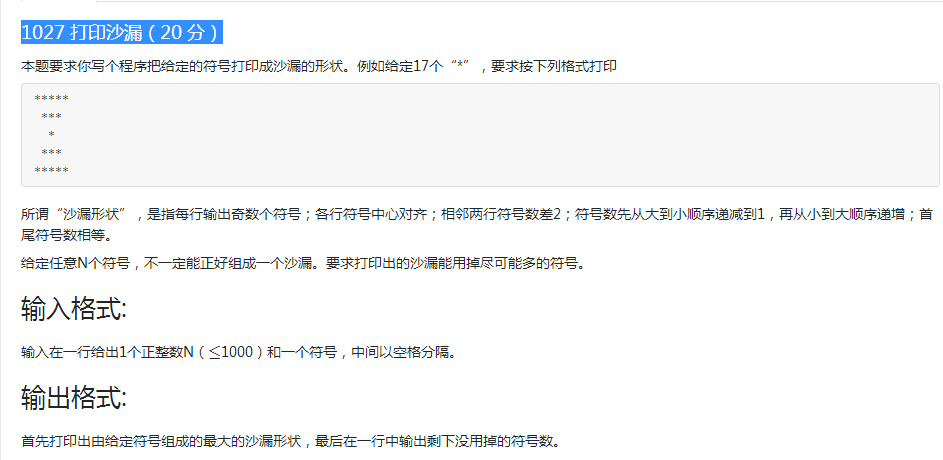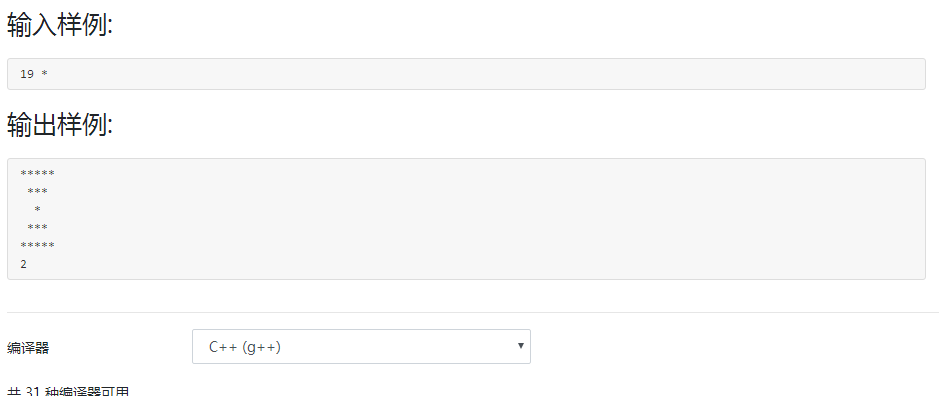C++ 1027 打印沙漏（20 分）``````#include<iostream>
using namespace std;

int main()
{
int a;
char b;
cin>>a>>b;
int k=0,n,sheng;

while(1){
k++;
if((2*k*k-1<=a)&&(2*(k+1)*(k+1)-1>a)){
n=k;
break;
}
}

sheng = a-2*n*n+1;//剩下没有用掉的数
for(int i=n;i>0;i--){
for(int j=0;j<n-i;j++){
cout<<" ";
}
for(int j=n-i;j<n+i-1;j++){
cout<<b;
}
for(int j=n+i-1;j<2*n-1;j++){
// cout<<" ";      // 右边没有空格  题目中也看不到说明  多捞啊 测试了好几遍才发现格式错误原因
}
cout<<endl;
}

for (int i=2;i<=n;i++){
for(int j=0;j<n-i;j++) cout<<" ";
for(int j=n-i;j<n+i-1;j++) cout<<b;
// for(int j=n+i-1;j<2*n-1;j++) cout<<" ";    // 右边没有空格  题目中也看不到说明  多捞啊 测试了好几遍才发现格式错误原因
cout<<endl;

}
cout<<sheng;
return 0;
}``````

0条评论